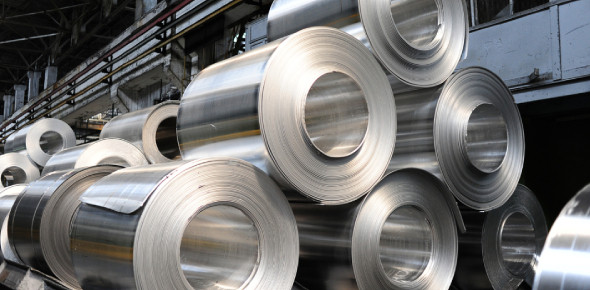# Aluminium Alloy Properties Quiz!

Approved & Edited by ProProfs Editorial Team
The editorial team at ProProfs Quizzes consists of a select group of subject experts, trivia writers, and quiz masters who have authored over 10,000 quizzes taken by more than 100 million users. This team includes our in-house seasoned quiz moderators and subject matter experts. Our editorial experts, spread across the world, are rigorously trained using our comprehensive guidelines to ensure that you receive the highest quality quizzes.
| By Gapher
G
Gapher
Community Contributor
Quizzes Created: 14 | Total Attempts: 6,367
Questions: 10 | Attempts: 215Settings.

• 1.

### Which of the following is the atomic number of Aluminium?

• A.

13

• B.

15

• C.

17

• D.

11

A. 13
Explanation
The atomic number of an element represents the number of protons in the nucleus of an atom. In the case of Aluminium, the atomic number is 13, which means that an Aluminium atom has 13 protons.

Rate this question:

• 2.

### By mass, aluminium makes up to how much of the earth's crust?

• A.

8%

• B.

15%

• C.

5%

• D.

10%

A. 8%
Explanation
Aluminium makes up approximately 8% of the earth's crust by mass. This means that out of all the elements and compounds that make up the earth's crust, about 8% of it is aluminium. This is a significant amount considering the vastness and complexity of the earth's crust. It also highlights the abundance of aluminium in the earth's composition.

Rate this question:

• 3.

### Amongst the abundant elements, Aluminium is of which position?

• A.

Second

• B.

Fifth

• C.

Third

• D.

Fourth

C. Third
Explanation
Aluminium is the third most abundant element on Earth. It is found in large quantities in the Earth's crust and is widely used in various industries due to its lightweight, corrosion resistance, and conductivity properties.

Rate this question:

• 4.

### Which of the following is the chief ore of aluminium?

• A.

Manganese

• B.

• C.

Anglesite

• D.

Bauxite

D. Bauxite
Explanation
Bauxite is the chief ore of aluminium. It is a sedimentary rock that is rich in aluminium minerals, primarily gibbsite, boehmite, and diaspore. Bauxite is formed through weathering of aluminium-rich rocks over millions of years. It is the main source of aluminium and is widely used in the production of aluminum metal, as well as in the manufacturing of various products such as cars, airplanes, electrical wiring, and packaging materials.

Rate this question:

• 5.

### It is found combined in how many different minerals?

• A.

Less than 250

• B.

Over 270

• C.

290

• D.

Over 280

B. Over 270
Explanation
The correct answer is "Over 270". This means that the mineral in question is found combined in more than 270 different minerals.

Rate this question:

• 6.

### Which of the following is the electrons per shell of Aluminium?

• A.

2, 8, 3

• B.

3,9,4

• C.

2, 9, 5

• D.

1, 8, 3

A. 2, 8, 3
Explanation
The correct answer is 2, 8, 3. This represents the number of electrons in each shell of an aluminium atom. The first shell can hold a maximum of 2 electrons, the second shell can hold a maximum of 8 electrons, and the third shell can hold a maximum of 3 electrons.

Rate this question:

• 7.

### What is the heat of vaporization?

• A.

284 kJ/mol

• B.

10.71 kJ/mol

• C.

198 kJ/mol

• D.

239 kJ/mol

A. 284 kJ/mol
Explanation
The heat of vaporization is the amount of heat energy required to convert one mole of a substance from its liquid phase to its gaseous phase at a constant temperature and pressure. In this case, the correct answer is 284 kJ/mol, which means that it takes 284 kilojoules of energy to vaporize one mole of the substance in question.

Rate this question:

• 8.

### Which of the following is the molar heat capacity?

• A.

127 kJ/mol

• B.

933 J/(mol.K)

• C.

24.20 J/(mol.K)

• D.

10.71 kJ/mol

C. 24.20 J/(mol.K)
Explanation
The molar heat capacity is a measure of the amount of heat energy required to raise the temperature of one mole of a substance by one degree Kelvin. In this case, the correct answer is 24.20 J/(mol.K), as it is expressed in units of joules per mole per Kelvin, which is the standard unit for molar heat capacity. The other options are expressed in units of kilojoules per mole, which is not the correct unit for molar heat capacity.

Rate this question:

• 9.

### Which of the following is the Pauling scale of aluminium?

• A.

2.56

• B.

1.61

• C.

1.90

• D.

1.85

B. 1.61
Explanation
The Pauling scale is a measure of electronegativity, which is the ability of an atom to attract electrons towards itself in a covalent bond. The electronegativity of aluminum on the Pauling scale is 1.61. This value indicates that aluminum has a moderate ability to attract electrons towards itself in a chemical bond.

Rate this question:

• 10.

### What is the Van der Waals radius of Aluminium?

• A.

189 pm

• B.

256 pm

• C.

157 pm

• D.

184 pm

D. 184 pm
Explanation
The Van der Waals radius of an atom represents the hypothetical radius of an atom when it is not bonded to any other atom. It is a measure of the size of the atom. In this case, the correct answer is 184 pm, which means that the Van der Waals radius of Aluminium is 184 picometers.

Rate this question:

Related TopicsBack to top